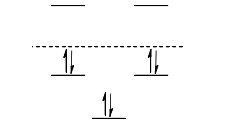# Problem: For the energy diagram shown below:  1) There are 3 antibonding orbitals and 2 bonding orbitals 2) There are 2 antibonding orbitals and 2 non-bonding orbitals and 1 bonding orbital 3) There are 2 antibonding orbitals and  3 bonding orbitals 4) There are 2 antibonding orbitals and 2 non-bonding orbitals and 1 bonding orbital 5) There is 1 bonding orbital and 4 antibonding orbitals

###### Problem Details

For the energy diagram shown below:

1) There are 3 antibonding orbitals and 2 bonding orbitals

2) There are 2 antibonding orbitals and 2 non-bonding orbitals and 1 bonding orbital

3) There are 2 antibonding orbitals and  3 bonding orbitals

4) There are 2 antibonding orbitals and 2 non-bonding orbitals and 1 bonding orbital

5) There is 1 bonding orbital and 4 antibonding orbitals